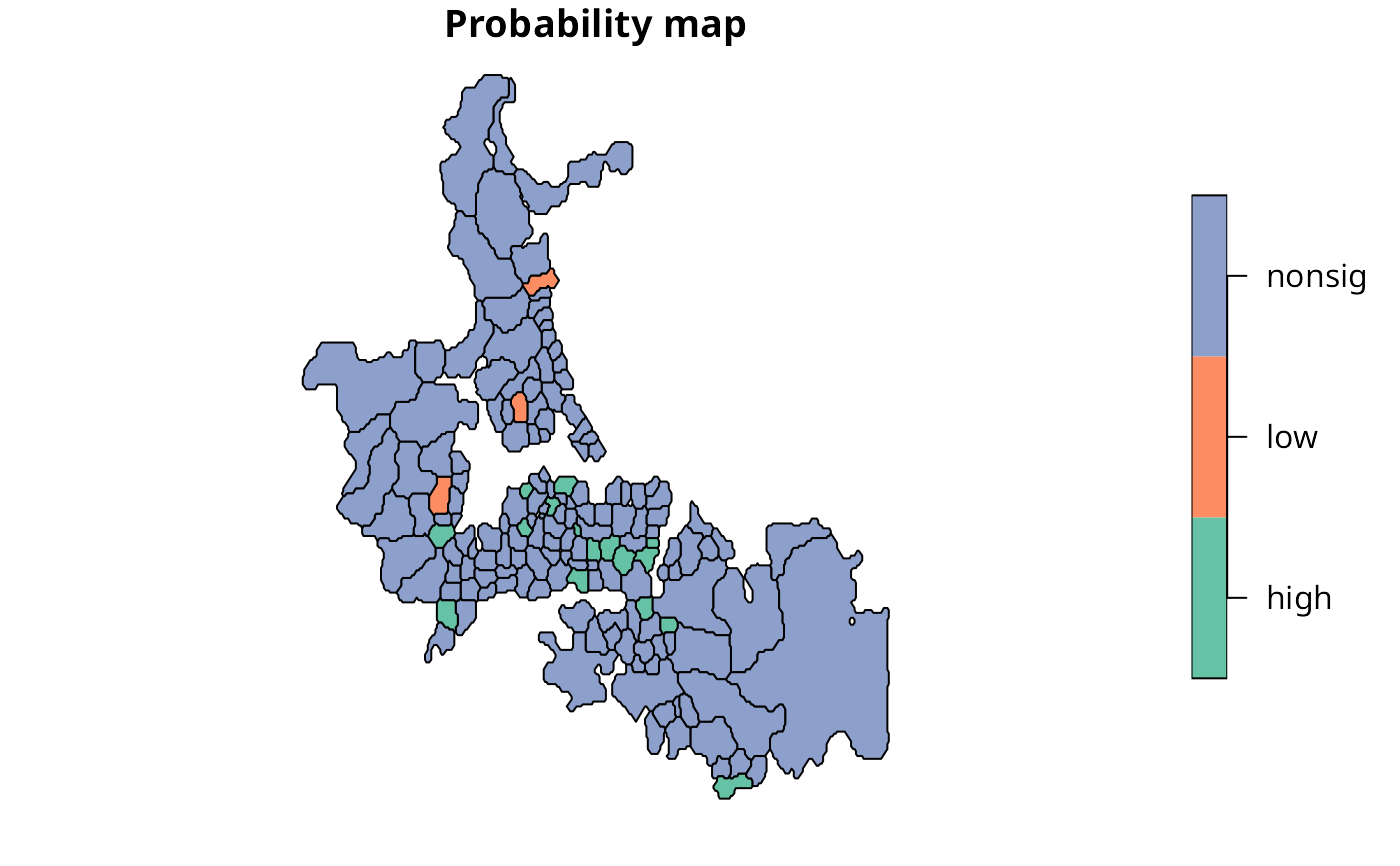Calculates Choynowski probability map values.

choynowski(n, x, row.names=NULL, tol = .Machine$double.eps^0.5, legacy=FALSE) ## Arguments n a numeric vector of counts of cases x a numeric vector of populations at risk row.names row names passed through to output data frame tol accumulate values for observed counts >= expected until value less than tol legacy default FALSE using vectorised alternating side ppois version, if true use original version written from sources and iterating down to tol ## Value A data frame with columns: pmap Poisson probability map values: probablility of getting a more extreme'' count than actually observed, one-tailed with less than expected and more than expected folded together type logical: TRUE if observed count less than expected ## Author Roger Bivand Roger.Bivand@nhh.no ## See also probmap ## Examples auckland <- st_read(system.file("shapes/auckland.shp", package="spData"), quiet=TRUE) auckland.nb <- poly2nb(auckland) res <- choynowski(auckland$M77_85, 9*auckland$Und5_81) resl <- choynowski(auckland$M77_85, 9*auckland$Und5_81, legacy=TRUE) all.equal(res, resl) #>  TRUE rt <- sum(auckland$M77_85)/sum(9*auckland$Und5_81) ch_ppois_pmap <- numeric(length(auckland$Und5_81))
side <- c("greater", "less")
for (i in seq(along=ch_ppois_pmap)) {
ch_ppois_pmap[i] <- poisson.test(auckland$M77_85[i], r=rt, T=(9*auckland$Und5_81[i]), alternative=side[(res$type[i]+1)])$p.value
}
all.equal(ch_ppois_pmap, res$pmap) #>  TRUE res1 <- probmap(auckland$M77_85, 9*auckland$Und5_81) table(abs(res$pmap - res1$pmap) < 0.00001, res$type)
#>
#>         FALSE TRUE
#>   FALSE    74    0
#>   TRUE      0   93
lt005 <- (res$pmap < 0.05) & (res$type)
ge005 <- (res$pmap < 0.05) & (!res$type)
cols <- rep("nonsig", length(lt005))
cols[lt005] <- "low"
cols[ge005] <- "high"
auckland\$cols <- factor(cols)
plot(auckland[,"cols"], main="Probability map")